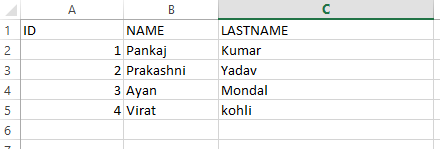GeeksforGeeks App
Open AppBrowser
Continue

# Reading and Writing Data to Excel File in Java using Apache POI

In Java, reading an Excel file is not similar to reading a Word file because of cells in an Excel file. JDK does not provide a direct API to read data from Excel files for which we have to toggle to a third-party library that is Apache POI. Apache POI is an open-source java library designed for reading and writing Microsoft documents in order to create and manipulate various file formats based on Microsoft Office. Using POI, one should be able to perform create, modify and display/read operations on the following file formats.
For Example, Java doesn’t provide built-in support for working with excel files, so we need to look for open-source APIs for the job. Apache POI provides Java API for manipulating various file formats based on the Office Open XML (OOXML) standard and OLE2 standard from Microsoft. Apache POI releases are available under the Apache License (V2.0).

### Writing an Excel File

Earlier we introduced Apache POI- a Java API useful for interacting with Microsoft Office documents. Now we’ll see how can we read and write to an excel file using the API.

Procedure: Writing a file using POI is very simple and involve the following steps:

1. Create a workbook
2. Create a sheet in the workbook
3. Create a row in the sheet
4. Add cells in the sheet
5. Repeat steps 3 and 4 to write more data.
6. Close the output stream.

Example:

## Java

 `// Java Program to Illustrate Writing ``// Data to Excel File using Apache POI`` ` `// Import statements``import` `java.io.FileOutputStream;``import` `java.io.IOException;``import` `org.apache.poi.ss.usermodel.Cell;``import` `org.apache.poi.ss.usermodel.Row;``import` `org.apache.poi.xssf.usermodel.XSSFSheet;``import` `org.apache.poi.xssf.usermodel.XSSFWorkbook;`` ` `// Main class``public` `class` `GFG {`` ` `    ``// Main driver method``    ``public` `static` `void` `main(String[] args)``    ``{`` ` `        ``// Blank workbook``        ``XSSFWorkbook workbook = ``new` `XSSFWorkbook();`` ` `        ``// Creating a blank Excel sheet``        ``XSSFSheet sheet``            ``= workbook.createSheet(``"student Details"``);`` ` `        ``// Creating an empty TreeMap of string and Object][]``        ``// type``        ``Map data``            ``= ``new` `TreeMap();`` ` `        ``// Writing data to Object[]``        ``// using put() method``        ``data.put(``"1"``,``                 ``new` `Object[] { ``"ID"``, ``"NAME"``, ``"LASTNAME"` `});``        ``data.put(``"2"``,``                 ``new` `Object[] { ``1``, ``"Pankaj"``, ``"Kumar"` `});``        ``data.put(``"3"``,``                 ``new` `Object[] { ``2``, ``"Prakashni"``, ``"Yadav"` `});``        ``data.put(``"4"``, ``new` `Object[] { ``3``, ``"Ayan"``, ``"Mondal"` `});``        ``data.put(``"5"``, ``new` `Object[] { ``4``, ``"Virat"``, ``"kohli"` `});`` ` `        ``// Iterating over data and writing it to sheet``        ``Set keyset = data.keySet();`` ` `        ``int` `rownum = ``0``;`` ` `        ``for` `(String key : keyset) {`` ` `            ``// Creating a new row in the sheet``            ``Row row = sheet.createRow(rownum++);`` ` `            ``Object[] objArr = data.get(key);`` ` `            ``int` `cellnum = ``0``;`` ` `            ``for` `(Object obj : objArr) {`` ` `                ``// This line creates a cell in the next``                ``//  column of that row``                ``Cell cell = row.createCell(cellnum++);`` ` `                ``if` `(obj ``instanceof` `String)``                    ``cell.setCellValue((String)obj);`` ` `                ``else` `if` `(obj ``instanceof` `Integer)``                    ``cell.setCellValue((Integer)obj);``            ``}``        ``}`` ` `        ``// Try block to check for exceptions``        ``try` `{`` ` `            ``// Writing the workbook``            ``FileOutputStream out = ``new` `FileOutputStream(``                ``new` `File(``"gfgcontribute.xlsx"``));``            ``workbook.write(out);`` ` `            ``// Closing file output connections``            ``out.close();`` ` `            ``// Console message for successful execution of``            ``// program``            ``System.out.println(``                ``"gfgcontribute.xlsx written successfully on disk."``);``        ``}`` ` `        ``// Catch block to handle exceptions``        ``catch` `(Exception e) {`` ` `            ``// Display exceptions along with line number``            ``// using printStackTrace() method``            ``e.printStackTrace();``        ``}``    ``}``}`

Procedure: Reading an excel file is also very simple if we divide this into steps.

1. Create workbook instance from excel sheet
2. Get to the desired sheet
3. Increment row number
4. iterate over all cells in a row
5. repeat steps 3 and 4 until all data is read.
6. Close the output stream.

Example:

## Java

 `// Java Program to Illustrate Reading``// Data to Excel File Using Apache POI`` ` `// Import statements``import` `java.io.File;``import` `java.io.FileInputStream;``import` `java.io.IOException;``import` `org.apache.poi.hssf.usermodel.HSSFSheet;``import` `org.apache.poi.hssf.usermodel.HSSFWorkbook;``import` `org.apache.poi.ss.usermodel.Cell;``import` `org.apache.poi.ss.usermodel.FormulaEvaluator;``import` `org.apache.poi.ss.usermodel.Row;`` ` `// Main class``public` `class` `GFG {`` ` `    ``// Main driver method``    ``public` `static` `void` `main(String[] args)``    ``{`` ` `        ``// Try block to check for exceptions``        ``try` `{`` ` `            ``// Reading file from local directory``            ``FileInputStream file = ``new` `FileInputStream(``                ``new` `File(``"gfgcontribute.xlsx"``));`` ` `            ``// Create Workbook instance holding reference to``            ``// .xlsx file``            ``XSSFWorkbook workbook = ``new` `XSSFWorkbook(file);`` ` `            ``// Get first/desired sheet from the workbook``            ``XSSFSheet sheet = workbook.getSheetAt(``0``);`` ` `            ``// Iterate through each rows one by one``            ``Iterator rowIterator = sheet.iterator();`` ` `            ``// Till there is an element condition holds true``            ``while` `(rowIterator.hasNext()) {`` ` `                ``Row row = rowIterator.next();`` ` `                ``// For each row, iterate through all the``                ``// columns``                ``Iterator cellIterator``                    ``= row.cellIterator();`` ` `                ``while` `(cellIterator.hasNext()) {`` ` `                    ``Cell cell = cellIterator.next();`` ` `                    ``// Checking the cell type and format``                    ``// accordingly``                    ``switch` `(cell.getCellType()) {`` ` `                    ``// Case 1``                    ``case` `Cell.CELL_TYPE_NUMERIC:``                        ``System.out.print(``                            ``cell.getNumericCellValue()``                            ``+ ``"t"``);``                        ``break``;`` ` `                    ``// Case 2``                    ``case` `Cell.CELL_TYPE_STRING:``                        ``System.out.print(``                            ``cell.getStringCellValue()``                            ``+ ``"t"``);``                        ``break``;``                    ``}``                ``}`` ` `                ``System.out.println(``""``);``            ``}`` ` `            ``// Closing file output streams``            ``file.close();``        ``}`` ` `        ``// Catch block to handle exceptions``        ``catch` `(Exception e) {`` ` `            ``// Display the exception along with line number``            ``// using printStackTrace() method``            ``e.printStackTrace();``        ``}``    ``}``}`

Output:Geeks, now you must be wondering what if we need to read a file at a different location, so the below example explains it all.

Example 1-A:

```// Java Program to Read a File From Different Location

// Getting file from local directory
private static final String FILE_NAME
= "C:\\Users\\pankaj\\Desktop\\projectOutput\\mobilitymodel.xlsx";

// Method
public static void write() throws IOException, InvalidFormatException
{

InputStream inp = new FileInputStream(FILE_NAME);
Workbook wb = WorkbookFactory.create(inp);
Sheet sheet = wb.getSheetAt(0);
........
}```

Example 1-B:

```// Reading and Writing data to excel file using Apache POI
// Via Appending to the Existing File

// Getting the path from the local directory
private static final String FILE_NAME
= "C:\\Users\\pankaj\\Desktop\\projectOutput\\blo.xlsx";

// Method
public static void write() throws IOException, InvalidFormatException
{

InputStream inp = new FileInputStream(FILE_NAME);
Workbook wb = WorkbookFactory.create(inp);
Sheet sheet = wb.getSheetAt(0);

int num = sheet.getLastRowNum();
Row row = sheet.createRow(++num);
row.createCell(0).setCellValue("xyz");
.....
..

// Now it will write the output to a file
FileOutputStream fileOut = new FileOutputStream(FILE_NAME);
wb.write(fileOut);

// Closing the file connections
fileOut.close();
}```